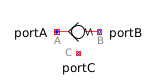Atan - MapleSim Help

Complex Atan

Output the arc tangent of the inputDescription The Complex Atan (or Atan) component computes the inverse-tangent of the complex input $u$. To use the complex conjugate of $u$, set Use Conjugate Input to $\mathrm{true}$.Equations $y=\mathrm{arctan}\left(u\right)$Connections

 Name Description Modelica ID $u$ Complex input signal u $y$ Complex output signal yParameters

 Name Default Units Description Modelica ID Use Conjugate Input $\mathrm{false}$ If true, use the complex conjugate of the input useConjugateInputModelica Standard Library The component described in this topic is from the Modelica Standard Library. To view the original documentation, which includes author and copyright information, click here.